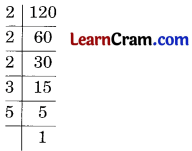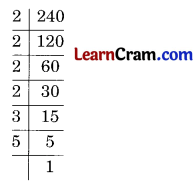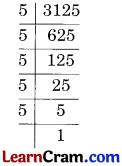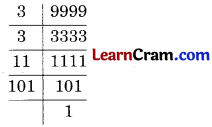# DAV Class 6 Maths Chapter 2 Worksheet 4 Solutions

The DAV Class 6 Maths Book Solutions Pdf and DAV Class 6 Maths Chapter 2 Worksheet 4 Solutions of Factors and Multiples offer comprehensive answers to textbook questions.

## DAV Class 6 Maths Ch 2 WS 4 Solutions

Question 1.
Find the prime factorisation of the following:

(a) 78
Solution:Prime factors of 78 = 2 × 3 × 13

(b) 120
Solution:Prime factors of 120 = 2 × 2 × 2 × 3 × 5

(c) 256
Solution:Prime factors of 256 = 2 × 2 × 2 × 2 × 2 × 2 × 2 × 2

(d) 84
Solution:Prime factors of 84 = 2 × 2 × 3 × 7(e) 441
Solution:Prime factors of 441 = 3 × 3 × 7 × 7

(f) 240
Solution:Prime factors of 240 = 2 × 2 × 2 × 2 × 3 × 5

(g) 2304
Solution:Prime factors of 2304 = 2 × 2 × 2 × 2 × 2 × 2 × 2 × 2 × 3 × 3

(h) 3125
Solution:Prime factors of 3125 = 5 × 5 × 5 × 5 × 5

(i) 1260
Solution:Prime factors of 1260 = 2 × 2 × 3 × 3 × 5 × 7Question 2.
Write the greatest 4 digit number. Express it as a product of primes.
Solution:
The greatest 4-digit number is 9999Product of prime factors = 3 × 3 × 11 × 101.

Question 3.
Write the smallest 4 digit number and show its prime factorisation.
Solution:
4-digit smallest number is 1000Prime factors of 1000 = 2 × 2 × 2 × 5 × 5 × 5.

Question 4.
Express each of the following numbers as sum of two odd primes:

(a) 18
Solution:
18 = 5 + 13

(b) 32
Solution:
32 = 13 + 19

(c) 66
Solution:
66 = 29 + 37

(d) 90
Solution:
90 = 53 + 37Question 5.
Express the following as sum of three odd primes:

(a) 41
Solution:
41 = 3 + 7 + 31

(b) 23
Solution:
23 = 3 + 7 + 13

(c) 75
Solution:
75 = 3 + 11 + 61

(d) 59
Solution:
59 = 3 + 13 + 43